##### Actions

A quadrature formula of highest algebraic accuracy of the type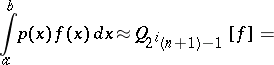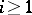, whereare the nodes of a Gauss quadrature formula and the nodes ofare fixed in the construction of[a2]. Nested sequences of Kronrod–Patterson formulas are used for the numerical approximation of definite integrals with practical error estimate, in particular in the non-adaptive routines of the numerical integration package QUADPACK [a4] and in the standard numerical software libraries.

The algebraic accuracy ofis at least. The free nodesof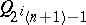are precisely the zeros of the polynomial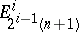which satisfieswhere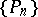is the system of orthogonal polynomials associated with,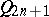is the Gauss–Kronrod quadrature formula, and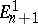is the Stieltjes polynomial (cf. Stieltjes polynomials). For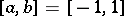and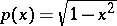,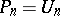, the Chebyshev polynomial of the second kind (cf. Chebyshev polynomials), and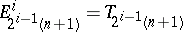, the Chebyshev polynomial of the first kind. In this case, all Kronrod–Patterson formulas are Gauss quadrature formulas (cf. Gauss quadrature formula). Hence, the algebraic accuracy ofis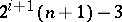, the nodes of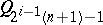andinterlace and the formulas have positive weights. Similar properties are known for the more general Bernstein–Szegö weight functions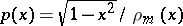, whereis a polynomial of degreewhich is positive on, see [a3].

Only very little is known for, which is the most important case for practical calculations. Tables of sequences of Kronrod–Patterson formulas have been given in [a2], [a4]. A numerical investigation forand Jacobi weight functions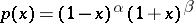,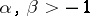, can be found in [a5].